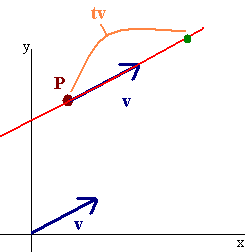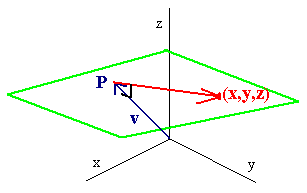# 1.6: Lines and Planes

$$\newcommand{\vecs}{\overset { \rightharpoonup} {\mathbf{#1}} }$$ $$\newcommand{\vecd}{\overset{-\!-\!\rightharpoonup}{\vphantom{a}\smash {#1}}}$$$$\newcommand{\id}{\mathrm{id}}$$ $$\newcommand{\Span}{\mathrm{span}}$$ $$\newcommand{\kernel}{\mathrm{null}\,}$$ $$\newcommand{\range}{\mathrm{range}\,}$$ $$\newcommand{\RealPart}{\mathrm{Re}}$$ $$\newcommand{\ImaginaryPart}{\mathrm{Im}}$$ $$\newcommand{\Argument}{\mathrm{Arg}}$$ $$\newcommand{\norm}{\| #1 \|}$$ $$\newcommand{\inner}{\langle #1, #2 \rangle}$$ $$\newcommand{\Span}{\mathrm{span}}$$ $$\newcommand{\id}{\mathrm{id}}$$ $$\newcommand{\Span}{\mathrm{span}}$$ $$\newcommand{\kernel}{\mathrm{null}\,}$$ $$\newcommand{\range}{\mathrm{range}\,}$$ $$\newcommand{\RealPart}{\mathrm{Re}}$$ $$\newcommand{\ImaginaryPart}{\mathrm{Im}}$$ $$\newcommand{\Argument}{\mathrm{Arg}}$$ $$\newcommand{\norm}{\| #1 \|}$$ $$\newcommand{\inner}{\langle #1, #2 \rangle}$$ $$\newcommand{\Span}{\mathrm{span}}$$$$\newcommand{\AA}{\unicode[.8,0]{x212B}}$$

## Lines

Our goal is to come up with the equation of a line given a vector v parallel to the line and a point (a,b,c) on the line. The figure (shown in 2D for simplicity) shows that if P is a point on the line then

$\langle x,y \rangle = P + tv\nonumber$

for some number $$t$$.The picture is the same for 3D. The formula is given below.

Parametric Equations of a Line

The parametric equations for the line through the point $$(a,b,c)$$ and parallel to the vector v are

$\langle x,y,z\rangle = \langle a,b,c\rangle + t\textbf{v}.\nonumber$

Example $$\PageIndex{1}$$

Find the parametric equations of the line that passes through the point $$(1, 2, 3)$$ and is parallel to the vector $$\langle 4, -2, 1\rangle$$.

Solution

We write:

$\langle x, y, z\rangle = \langle 1, 2, 3\rangle + t \langle 4, -2, 1\rangle = \langle 1 + 4t, 2 - 2t, 3 + t\rangle \nonumber$

or

$x(t) = 1 + 4t,\nonumber$

$y(t) = 2 - 2t,\nonumber$

$z(t) = 3 + t.\nonumber$

Exercise $$\PageIndex{1}$$

Find the parametric equations of the line through the two points $$(2,1,7)$$ and $$(1,3,5)$$.

Hint: a vector parallel to the line has tail at $$(2,1,7)$$ and head at $$(1,3,5)$$.

## Planes

If S is a plane then a vector n is normal (perpendicular) to the plane if it is orthogonal to every vector that lies on the plane. Suppose that n is a normal vector to a plane and $$(a,b,c)$$ is a point on the plane. Let $$(x,y,z)$$ be a general point on the plane, then

$\langle x - a, y - b, z - c\rangle \nonumber$

is parallel to the plane, hence

$\vec{n} \cdot \langle x - a, y - b, z - c\rangle = 0.\nonumber$

This defines the equation of the plane.Example $$\PageIndex{2}$$

Find the equation of the plane that contains the point $$(2,1,0)$$ and has normal vector $$\langle 1,2,3\rangle$$.

Solution

We have

$\langle 1,2,3\rangle \cdot \langle x - 2,y - 1,z - 0\rangle = 0\nonumber$

so that

$1(x - 2) + 2(y - 1) + 3z = 0\nonumber$

or

$x + 2y + 3z = 4.\nonumber$

Example $$\PageIndex{3}$$

Find the equation of the plane through the points

• $$P = (0,0,1)$$
• $$Q = (2,1,0)$$
• $$R = (1,1,1)$$

Solution

Let

$\textbf{v} = Q-P = \langle 2,1,-1\rangle \nonumber$

and

$\textbf{w} = R-P = \langle 1,1,0\rangle \nonumber$

then to find a vector normal to the plane, we find the cross product of $$v$$ and $$w$$:

$v \times w = \begin{vmatrix} \hat{\textbf{i}} & \hat{\textbf{i}} & \hat{\textbf{i}} \\ 2 & 1 &-1 \\ 1 &1 0 \end{vmatrix} = \hat{\textbf{i}} - \hat{\textbf{j}} + \hat{\textbf{k}} \nonumber$

or

$\langle 1, -1, 1\rangle .\nonumber$

We can now use the formula:

$\langle 1, -1, 1\rangle \cdot \langle x, y, z - 1\rangle = 0\nonumber$

or

$x - y + z - 1 = 0\nonumber$

or

$x - y + z = 1\nonumber$

## Distance Between a Point and a Plane

Let P be a point and Q be a point on a plane with normal vector n, then the distance between P and the plane is given by

Distance Between a Point $$P$$ and a Plane With Normal Vector n

Let $$Q$$ be a point on the plane with normal vector $$\vec{n}$$. The the distance from the point $$P$$ to this plane is given by

$Proj_nPQ = \dfrac{ ||PQ \cdot \vec{n}|| }{ || \vec{n}|| }.\nonumber$

Example $$\PageIndex{4}$$

Find the distance between the point $$(1,2,3)$$ and the plane

$2x - y - 2z = 5.\nonumber$

Solution

The normal vector can be read off from the equation as

$\vec{n} = \langle 2, -1, -2\rangle .\nonumber$Now find a convenient point on the plane such as $$Q = (0, -5, 0)$$. We have

$Q = \langle -1, -7, -3\rangle \nonumber$

and

$\vec{n} \cdot PQ = -2 + 7 + 6 = 11.\nonumber$

We find the magnitude of n by taking the square root of the sum of the squares. The sum is

$4 + 1 + 4 = 9\nonumber$

so

$|| \vec{n} || = 3.\nonumber$

Hence the distance from the point to the plane is $$\frac{11}{3}$$.

## The Angle Between Two Planes

The angle between two planes is given by the angle between the normal vectors.

Example $$\PageIndex{5}$$

Find the angle between the two planes

$3x - 2y + 5z = 1\nonumber$

and

$4x + 2y - z = 4.\nonumber$

We have the two normal vectors are

$\vec{n} = \langle 3,-2,5\rangle \nonumber$

and

$\vec{m} = \langle 4,2,-1\rangle .\nonumber$

We have

$\vec{n} \cdot \vec{ m} = 3\nonumber$

$|| \vec{n} || = \sqrt{38}\nonumber$

$|| \vec{m} || = \sqrt{21}\nonumber$

hence the angle is

$\cos^{-1} \left(\dfrac{3}{\sqrt{38}\sqrt{21}} \right) = 1.46 \, rad.\nonumber$